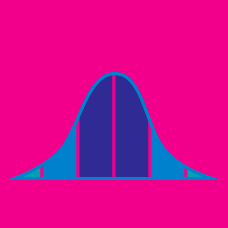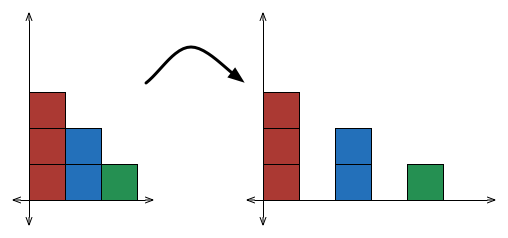Probability

# Variance - Definition

What is the variance of the following probability distribution: $\begin{array}{c}&P(X = -1) = 0.5, &P(X = 0) = 0.2, &P( X = 1) = 0.3 ?\end{array}$When all values of a dataset are doubled, what happens to the variance?

You throw a coin twice. The prize is $50$ dollars if it lands heads, and $4$ dollars if it is tails. If $X$ is the total prize you will end up receiving, then what is the variance of $X ?$

The probability distribution of a random variable $X$ is $\begin{array}{c}&P(X = 1) = a, &P(X = 2) = b, &P( X = 3) = 0.4. \end{array}$ If $a \cdot b=0.05$ and $a \geq b,$ then what is the variance of $X?$

The probability distribution of a random variable $X$ is $\begin{array}{c}&P(X = 1) = 0.1, &P(X = 2) = 0.2, &P( X = 3) = a.\end{array}$ What is the variance of $X?$

×